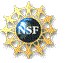# Robotics Laboratory

## Dexterous Robotic Cutting

Translation: Norwegian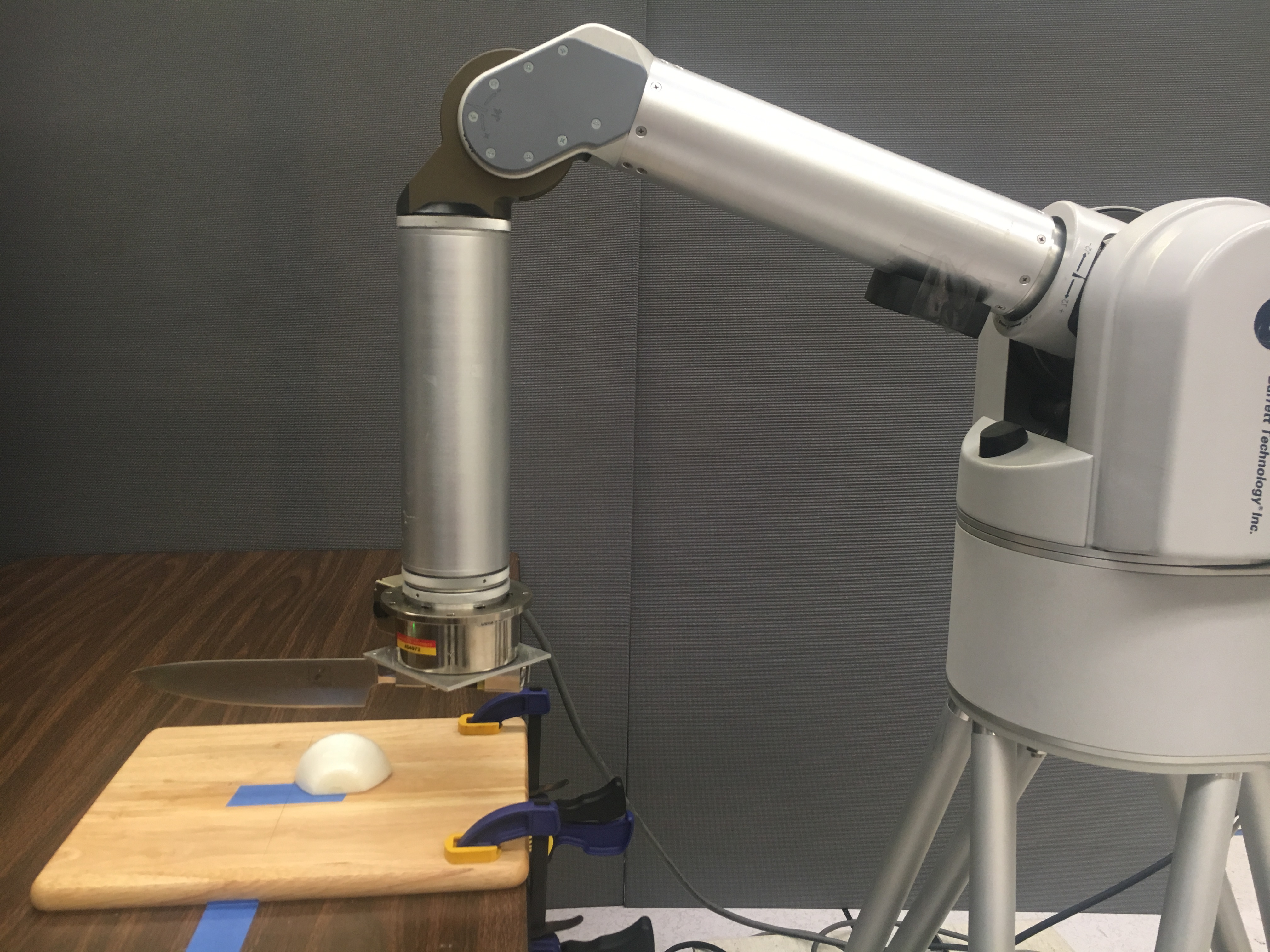Home robots have long been a fascination to the public. They are at the core of the quality of life technology, carrying high promises for relieving people from daily chores, and providing costeffective health care to the growing elderly population and people with disabilities. Automation of kitchen skills is an important part of home robotics, and also one of the ultimate tests for robots to achieve human-like dexterity. Despite its significance and appeal, until today robotic kitchen assistance has been limited to dish washing and sorting, and to cooking of food items prepared by human.

In this work, a 2-DOF robotic arm equipped with a force/torque sensor is used to cut through an object in a sequence of three moves: press, push, and slice. For each move, a separate control strategy in the Cartesian space is designed to incorporate contact and/or force constraints while following some prescribed trajectory.

### Mechanics and Control of Knife Motion

During cutting, each infinitesimal elememt on the knife receives a force $$d{\bf f} = d{\bf f}_C + d{\bf f}_U + d{\bf f}_F$$ from the object. The work done by $$-d{\bf f}_C$$ yields new fracture in the object, that by $$-d{\bf f}_U$$ causes an increase (or decrease) in the object’s strain energy, and that by $$-d{\bf f}_F$$ is dissipated through friction. Some foods like potatos and yams barely defrom during cutting, thus an assumption $$d{\bf f}_U$$ = 0 can be applied.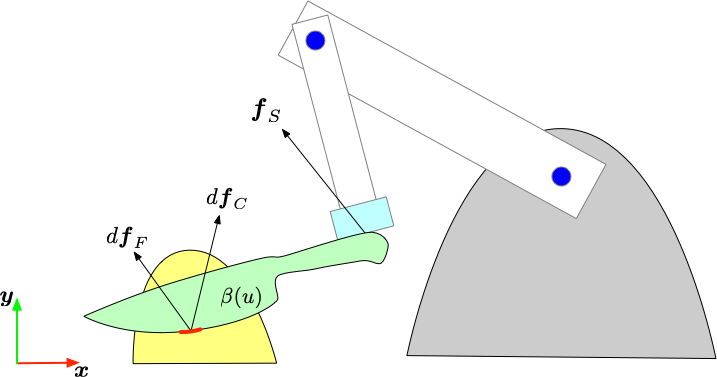The fracture force and frictional force can be calculated as follows: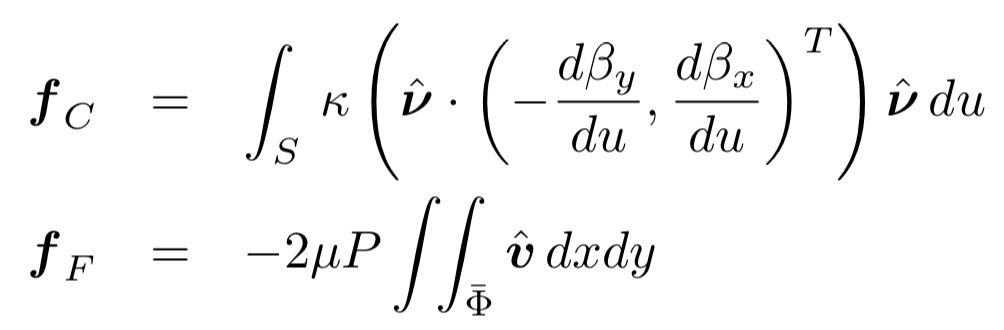in which, the integral interval $$S$$ is the knife blade that contact with the object and $$\Phi$$ is the cross secction between knife and object. $$\mu$$, $$\kappa$$, and $$P$$ are conefficient of friction, fracture toughness, and pressure distribution, respectively. $$(\beta_x, \beta_y)^T$$ describes the knife's balde curve in world frame, while $$u$$ is the local $$x$$-cooridinate for the knife blade.

Phase Contorller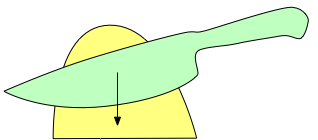Press
• Position control in y-direction to achieve certain frature rate
• Force control to keep constant knife orientation
$${\bf \tau} = ML_a \left(\ddot{y}_d + k_v \dot{y}_e + k_p y_e + k_i \int y_e dt\right) + N_a + F_a$$     where $$F_a = (h + k_{fd} \dot{h} + k_{fi} \int hdt) \nabla h^T$$     is a generalized constraint force such as rotation of the knife.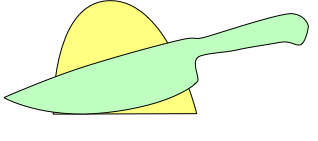Push
• Force control in y-direction until the knife-board contact force reaches a desired level
$${\bf \tau} = N_a + F_a - J_c^T \left(0,\, f_d + \bar{k}_{fd} \dot{f}_e + \bar{k}_{fi} \int f_e dt\right)^T$$     where $$F_a = (h + k_{fd} \dot{h} + k_{fi} \int hdt) \nabla h^T$$    same as the pressing phase.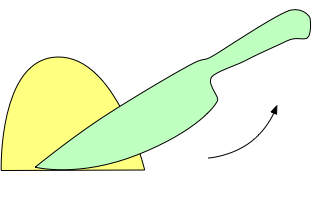Slice
• Position control in x-direction to achieve steady cutting progress
• Force control in y-direction to maintain the contact force level
$${\bf \tau} = ML_c\left(\bar{k}_v\dot{x}_e + \bar{k}_p x_e + \bar{k}_i \int x_e dt\right) + N_c + F_c$$     where $$F_c = -J_c^T \left(0,\, f_d + \bar{k}_{fd}\dot{f}_e + \bar{k}_{fi} \int f_e dt\right)^T$$
In the three controllers, $$M$$ is the mass matrix, $$L_a$$ and $$L_c$$ are the Jacobian for coordinate transformation, and $$N_a$$ and $$N_c$$ include the Coriolis, centrifugal, and gravity effects, and the external wrench.

A WAM arm equipped with a 6-axis F/T sensor was used in our experiment. We fitted the knife blade and spine by polynomials which were used to track the lowest point on the knife and the cross section between knife and object. The contour of the object was fitted over the points (close enough to the cutting plane) obtained by a Microsoft Kinect sensor. We also measured the coefficient of frciton, fracture toughness, and pressure distrabution for potato and onion to calculate the fracture force and frictional force.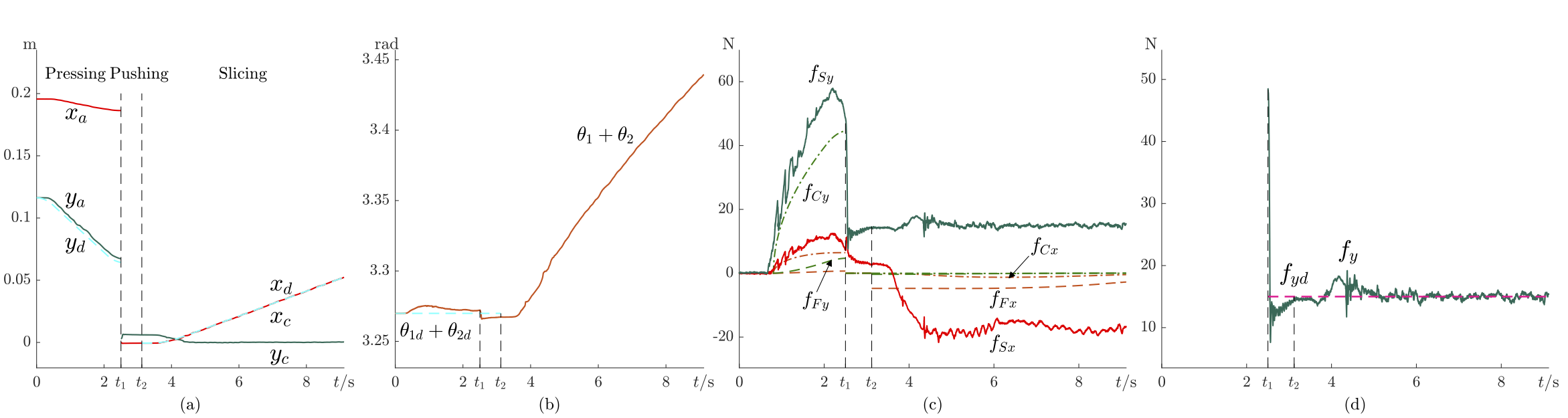The figure above shows the experimental results from cutting an onion. Each of (a)–(d) illustrates all three phases of cutting. In (a), the ordinate $$y_a$$ of the arm’s free end follows the desired trajectory $$y_d$$ during pressing, and the abscissa $$x_c$$ of the knife-board contact follows the desired trajectory $$x_d$$. In (b), the orientation $$\theta_1 + \theta_2$$ of the arm frame (hence that of the knife) stays almost constant in the first two phases, but increases in the third phase. In (c), the modeled frictional force $${\bf f}_F$$ and fracture force $${\bf f}_C$$ add up close to the sensed force $${\bf f}_S$$ in the pressing phase, except every time the knife enters a new layer in the onion. In the slicing phase, the modeled force in the $$y$$-direction is very small, since every point on the knife is moving almost in the $$x$$-direction. In (d), a sudden change in the contact force has been caused by a large gap between the desired force and actual force. The contact force converges quickly to the desired value and varies within a small range. Results from these experiments and more can be seen in the YouTube video at the top of the page (link).

### Modeling of Cutting Based on Fracture Mechanics and FEM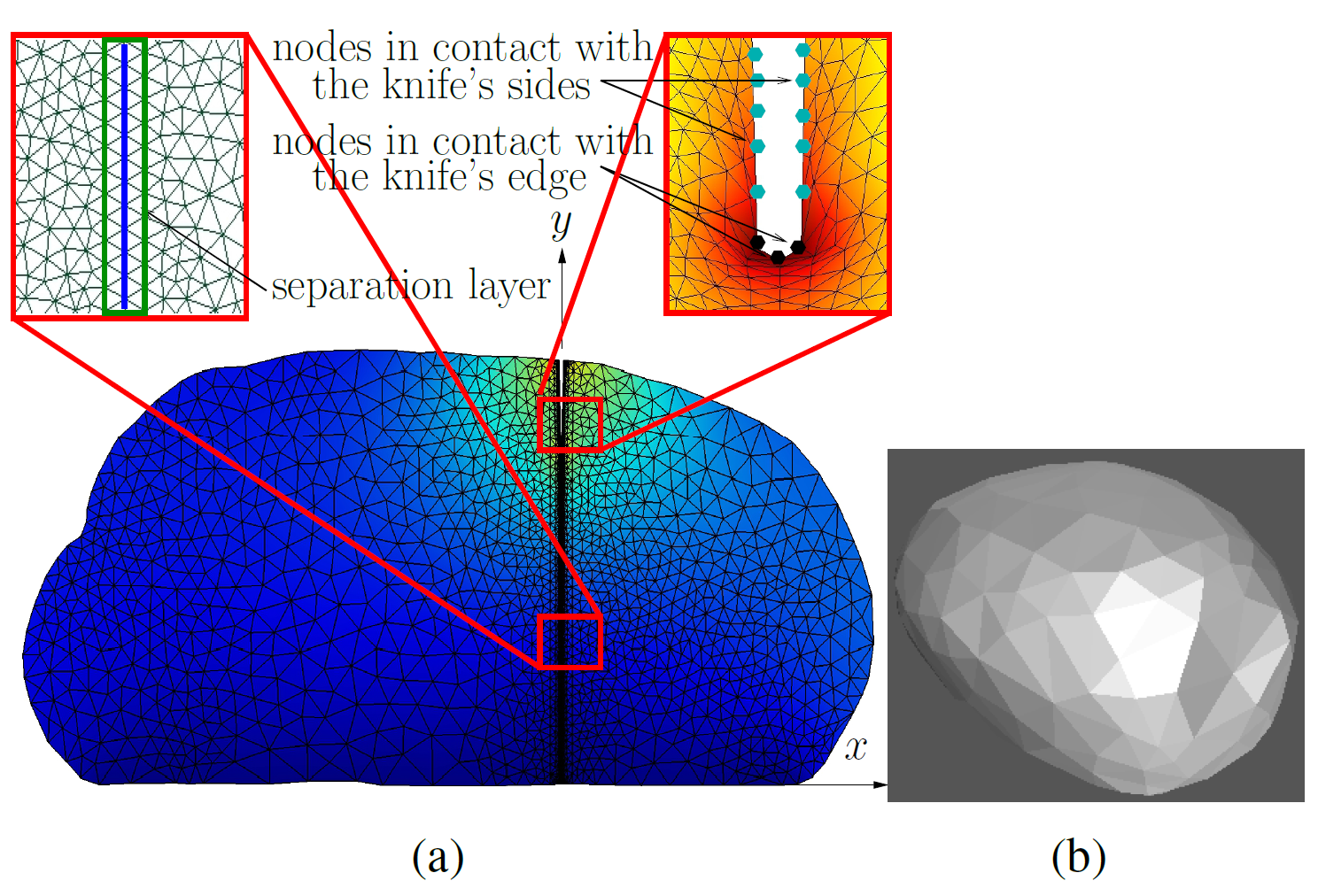Modeling of fracture and deformation during a cutting action, often based on the finite element method (FEM), provides the force and shape information used in knife control to implement a skill such as slice, chop, or dice. However, an object’s 3D mesh model can be computationally prohibitive for achieving a desired accuracy since numerous tiny elements must be used near the knife’s moving edge. To address this issue, we represent the object as evenly spaced slices normal to the cutting plane such that the 3D modeling task is reduced to multiple 2D modeling tasks. Each task is solvable via the same FEM procedure, and fracture and force can be then interpolated between every two adjacent slices. Experiment with an Adept arm and an ATI force/torque (F/T) sensor conducted over five food items has demonstrated good matches between the modeled force, torque, and work experienced by the knife, and the measurements by a six-axis force/torque sensor.